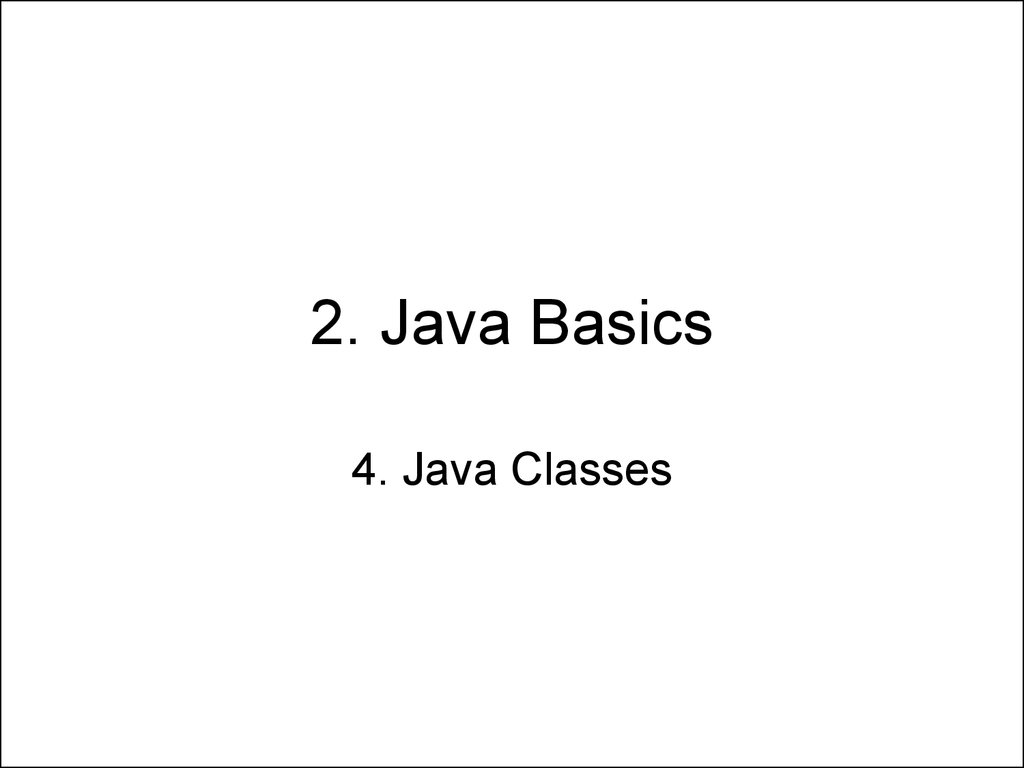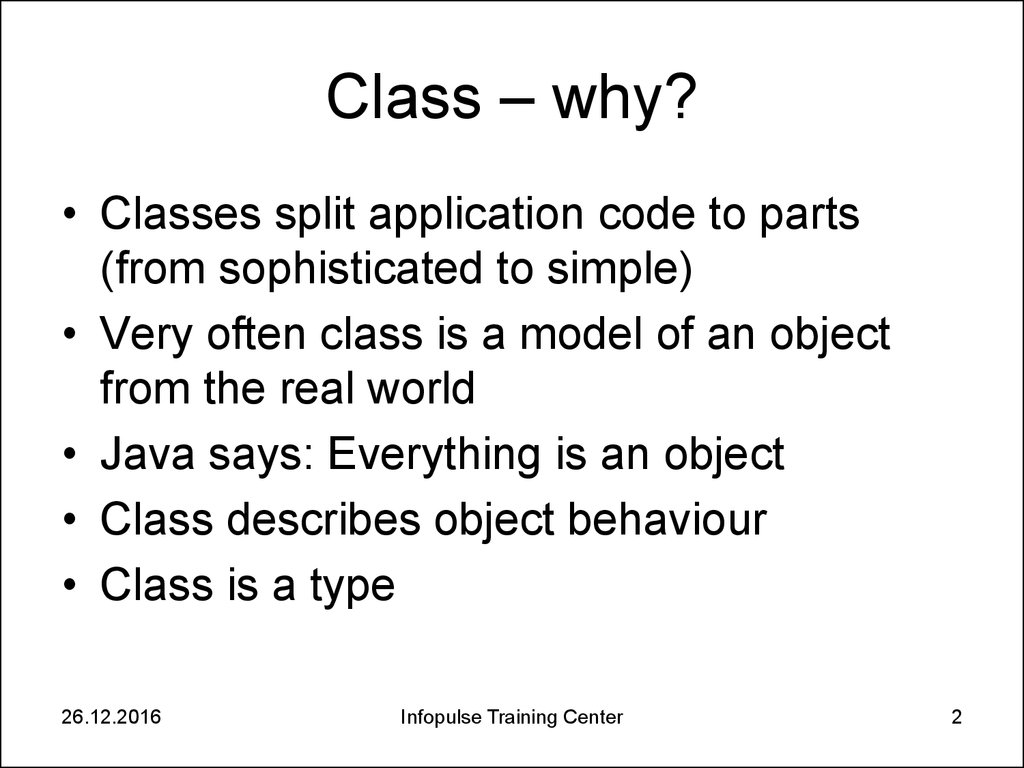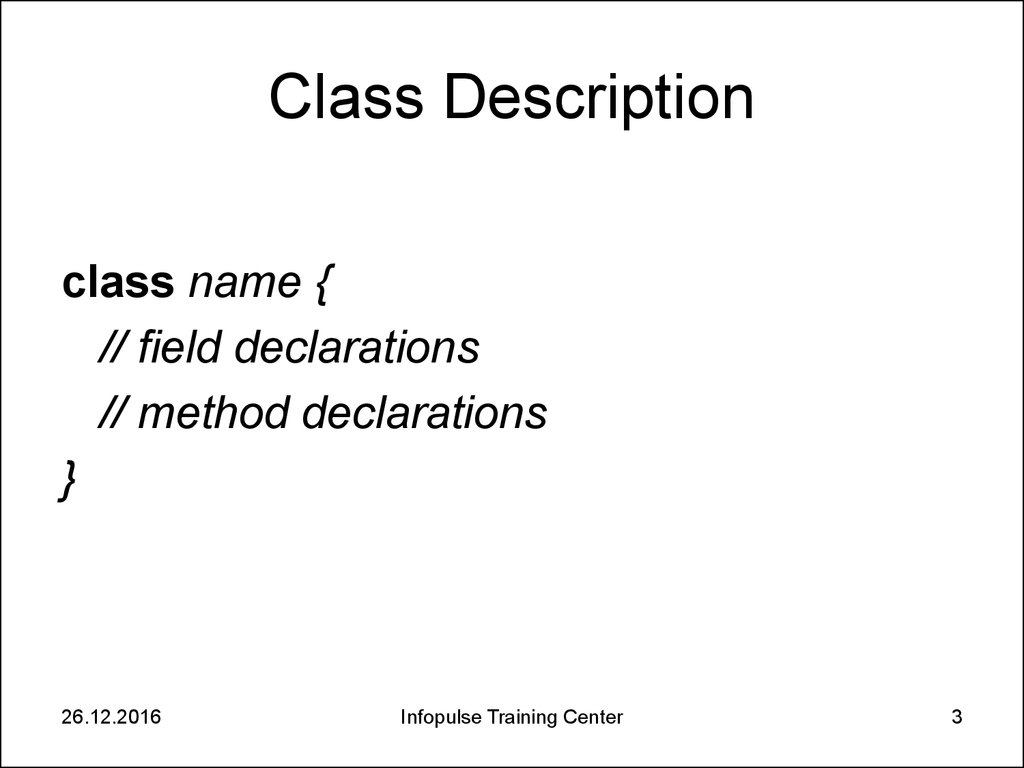2. Java Basics. 4. Java Classes

4. Java Classes

2. Class – why?

• Classes split application code to parts
(from sophisticated to simple)
• Very often class is a model of an object
from the real world
• Java says: Everything is an object
• Class describes object behaviour
• Class is a type
26.12.2016
Infopulse Training Center
2

3. Class Description

class name {
// field declarations
// method declarations
}
26.12.2016
Infopulse Training Center
3

4. Class Fields

• Class fields should be declared inside
class out of all class methods
• Fields can have primitive type, or
reference type such as array or object
• Fields are visible to all instance methods
• Fields are automatically initialized
(reference types with null, number types
with zero, boolean – with false)
26.12.2016
Infopulse Training Center
4

5. Defining Methods

return_type method_name (parameter_list){
// method body
}
Example:
int getFinalData(int a, int r){
int b = r % 18;
return a * 2 + b;
}
26.12.2016
Infopulse Training Center
5

6. Return Type

• The return type describes the value that
comes back from the method
• A method can have void return type
• Any method that is not declared void must
contain a return statement with a
corresponding return value
• Return statements for void return type is
not necessary
26.12.2016
Infopulse Training Center
6

7. Parameters

• Any data type is possible for a parameter of a
method
• Construct varargs is used to pass an arbitrary
number of values (e.g. type... args)
• Varargs can be used only in the final argument
position
• Parameters are passed into methods by value.
• The values of the object's fields can be
changed in the method
26.12.2016
Infopulse Training Center
7

8. Constructors

• Constructor name should be the same as class
name
• Constructor has no return type
• The compiler automatically provides a noargument, default constructor for any class
without parameters – don’t use this possibility,
declare such constructor explicitly
• A class can have several constructors (with
different sets of parameters)
26.12.2016
Infopulse Training Center
8

9. Objects

• Creating Object:
class_name object_variable = new construtor_call;
• Declaring a Variable to Refer to an Object:
class_name object_variable;
• Calling an Object's Methods:
object_variable.methodName(argumentList);
26.12.2016
Infopulse Training Center
9

10. Using the this Keyword

• this is a reference to the current object
• The most common example:
class Point {
int x = 0;
int y = 0;
//constructor
Point(int x, int y) {
this.x = x;
this.y = y;
}
}
26.12.2016
Infopulse Training Center
10

11. Complex Numbers (1 of 4)

• Is it always possible to solve square
2
ax
bx c 0 within real
equation
numbers set?
26.12.2016
Infopulse Training Center
11

12. Complex Numbers (2 of 4)

• Is it always possible to solve square
2
equation ax bx c 0 within real numbers
set?
• No, if b 2 4ac 0 it is impossible.
• We can expand real number set to complex
number set introducing new number type complex unit i - in such a way:
i * i = -1
26.12.2016
Infopulse Training Center
12

13. Complex Numbers (3 of 4)

• Number of a + b * i type where a and b are
real is called complex number.
• Every square equation can be solved
within complex numbers set.
• Moreover, every algebraic equation (with
arbitrary power) always can be solved
within complex numbers set.
26.12.2016
Infopulse Training Center
13

14. Complex Numbers (4 of 4)

• To add complex numbers use formula
(a1 b1i) (a2 b2i) (a1 a2 ) (b1 b2 )i
• To multiply complex numbers use formula
(a1 b1i) * (a2 b2i) (a1a2 b1b2 ) (a1b2 a2b1 )i
• To find absolute value of complex number
use formula r a 2 b 2
26.12.2016
Infopulse Training Center
14

15. Exercise 2.4.1.

• Create a class for saving and manipulating
complex numbers.
26.12.2016
Infopulse Training Center
15

16. Home Exercise 2.4.2 (1 of 2)

• 3D Vector is ordered sequence of 3
numbers (vector’s coordinates):
r ( x1 , x2 , x3 )
• Sum of two vectors
r 1 ( x11 , x12 , x13 )
r 2 ( x21 , x22 , x23 )
is a vector
r r1 r2 ( x11 x21 , x12 x22 , x13 x23 )
26.12.2016
Infopulse Training Center
16

17. Home Exercise 2.4.2 (2 of 2)

• Scalar product of two vectors
r 1 ( x11 , x12 , x13 )
r 2 ( x21 , x22 , x23 )
p x11 x21 x12 x22 x13 x23
is a number
• Vector product of two vectors is a vector
r r1 r2 ( x12 x23 x13 x22 , x13 x21 x11 x23 , x11 x22 x12 x21 )
• Vector’s module is a number m x12 x22 x32
• You should create a class Vector3D for
vector saving and manipulating
26.12.2016
Infopulse Training Center
17